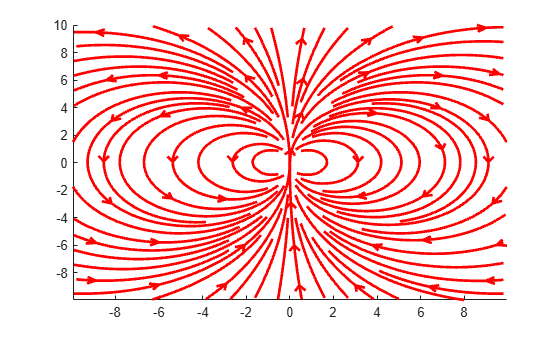streamline

Plot streamlines from 2-D or 3-D vector data

•Description

example

streamline(X,Y,Z,U,V,W,startx,starty,startz) draws streamlines from 3-D vector data U, V, W. Specify X, Y, and Z as the coordinate data. Specify startx,starty, and startz as the starting positions of the streamlines.

streamline(U,V,W,startx,starty,startz) uses the default coordinate data for U, V, and W. The (x,y,z) location for each element in U, V, and W is based on the column, row, and page index, respectively.

example

streamline(X,Y,U,V,startx,starty) draws streamlines from 2-D vector data U and V. Specify X and Y as the coordinate data. Specify startx and starty as the starting positions of the streamlines.

streamline(U,V,startx,starty) uses the default coordinate data for  U and V. The (x,y) location for each element in U and V is based on the column and row index, respectively.

example

streamline(verts) draws streamlines from vertices, specified as a cell array of vertex arrays (as returned by stream2, stream3, or streamslice).

streamline(___,options) draws streamlines using the specified options, defined as a one- or two-element vector with the form [step] or [step, maxvert], where step is the step-size for interpolating the vector data and maxvert is the maximum number of vertices in a streamline. Use this option with any of the input arguments from the previous syntaxes.

streamline(ax,___) plots into the specified axes, instead of into the current axes object (gca).

example

lineobj = streamline(___) returns a Line object. Use lineobj to modify properties of the streamlines after creating them. For a list of properties, see Line Properties.

Examples

collapse all

Load the wind dataset, which loads the 3-D arrays u, v, w, x, y, and z, representing air currents over regions of North America. Plot streamlines that start at evenly spaced points on the plane x = 80.

[startx,starty,startz] = meshgrid(80,20:10:50,0:5:15);
streamline(x,y,z,u,v,w,startx,starty,startz)
axis tight
view(3);Define arrays x, y, u, and v. Create a quiver plot to visualize the vector data. Then, plot streamlines that start at different points along the line y = 1.

[x,y] = meshgrid(0:0.1:1);
u = x;
v = -y;
quiver(x,y,u,v)
startx = 0.1:0.1:1;
starty = ones(size(startx));
streamline(x,y,u,v,startx,starty)Load the wind dataset and use stream3 to compute the vertices of streamlines that start at evenly spaced points on the plane x = 80. Then, plot the streamlines from the vertex data.

[startx,starty,startz] = meshgrid(80,20:10:50,0:5:15);
verts = stream3(x,y,z,u,v,w,startx,starty,startz);
streamline(verts)
axis tight
view(3);Plot streamlines and return the line objects in l.

[x,y] = meshgrid(-10:10);
u = 2.*x.*y;
v = y.^2 - x.^2;
l = streamslice(x,y,u,v);
axis tightSet the line width of the streamlines to 2. Set their color to red. Use the set function to change the properties of all the lines at the same time.

set(l,'LineWidth',2)
set(l,'Color','r');Input Arguments

collapse all

x-axis coordinate data, specified as a 2-D or 3-D array. It must be monotonic, but does not need to be uniformly spaced.

X, Y, Z, U, V, and W must be the same size.

You can use the meshgrid function to create X, Y, and Z matrices.

y-axis coordinate data, specified as a 2-D or 3-D array. It must be monotonic, but does not need to be uniformly spaced.

X, Y, Z, U, V, and W must be the same size.

You can use the meshgrid function to create X, Y, and Z matrices.

z-axis coordinate data, specified as a 3-D array. It must be monotonic, but does not need to be uniformly spaced.

X, Y, Z, U, V, and W must be the same size.

You can use the meshgrid function to create X, Y, and Z matrices.

x-components of vector data, specified as a 2-D or 3-D array.

X, Y, Z, U, V, and W must be the same size.

y-components of vector data, specified as a 2-D or 3-D array.

X, Y, Z, U, V, and W must be the same size.

z-components of vector data, specified as a 3-D array.

X, Y, Z, U, V, and W must be the same size.

x-axis streamline starting positions, specified as a vector or matrix.

startx, starty, and startz must be the same size.

y-axis streamline starting positions, specified as a vector or matrix.

startx, starty, and startz must be the same size.

z-axis streamline starting positions, specified as a vector or matrix.

startx, starty, and startz must be the same size.

Streamline vertices, specified as a cell array (as returned by stream2, stream3, or streamslice). Each element of the cell array is a matrix of vertices for one line.

Streamline drawing options, specified as a one- or two-element vector with the following form:

• [step]

• [step,maxvert]

Where step is the step-size used for interpolating the vector data and maxvert is the maximum number of vertices in a streamline.

The default step-size is .1 and the default maximum number of vertices is 1000.

Target axes, specified as an Axes object. If you do not specify the axes, then the streamline function uses the current axes.